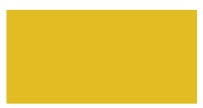Home | | Maths 4th Std | Properties of 3−D objects

# Properties of 3−D objects

A plane 2−dimensional shape that can be folded to form a 3−dimensional shape is called a net.

Properties of 3−D objects

Create 3D objects using clay and paper folding.

A plane 2−dimensional shape that can be folded to form a 3−dimensional shape is called a net. Nets are used to make floor maps of houses, layout planes of buildings, bridges and so on.

CubeA cube has 6 plane faces, 12 edges and 8 vertices. All the six faces are equal.

Examples

Dice, ice cubes, building blocks, rubiks

CuboidA cuboid has 6 plane faces. 12 edges and 8 vertices. Its opposite faces are equal.

Examples

Match box, bricks, eraser, book, toothpaste box.

SphereA sphere has only one curved surface. It has no vertices and edges.

Examples

ConeA cone has one plane face and one curved surface. It has one vertex.

Examples

Cone ice cream, Joker‛s cap

CylinderA cylinder has 2 plane faces and 1 curved surface.

It has no edges and vertices.

Examples

straw, gas cylinder, pipe.

Activities

a. Fold and form cube from the following netsb. Use these nets to form cuboids.c. Make a cone with semicircle.d. Make a cylinder using rectangle sheet.Try it

Make 3−D shapes using clay. (Individual Activity)Tags : Geometry | Term 1 Chapter 1 | 4th Maths , 4th Maths : Term 1 Unit 1 : Geometry
Study Material, Lecturing Notes, Assignment, Reference, Wiki description explanation, brief detail
4th Maths : Term 1 Unit 1 : Geometry : Properties of 3−D objects | Geometry | Term 1 Chapter 1 | 4th Maths